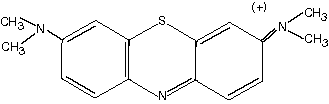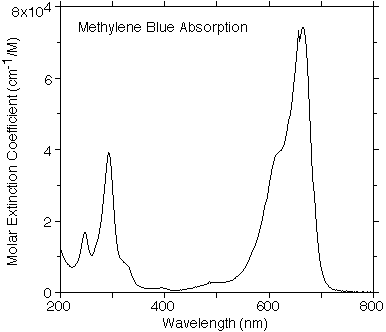### General PropertiesC16H18ClN3S, MW 319.85. Also called Swiss blue. One gram dissolves in about 25 ml of water, or in 65 ml alcohol. Peak absorption at 668 and 609 nm. --- Merck Index

### Uses

Used as a stain in bacteriology and as an oxidation-reduction indicator. An antidote to cyanide. Veterinary use as antiseptic.

Methylene Blue can be activated by light to an excited state which in turn activates oxygen to yield oxidizing radicals. Such radicals can cause crosslinking of amino acid residues on proteins and hence achieve some degree of crosslinking. We are investigating the use of MB to achieve photochemical welding of tissues.

### OMLC Methylene Blue Data

This spectrum was taken with a HP spectrophotometer using a 1 cm quartz cuvette filled with 10µM solution of Methylene Blue in Water by Scott Prahl.Tabulated data for the extinction coefficeint of methylene blue versus wavelength.

### The Molar Extinction Coefficient Defined

If the transmission through a sample is given by T, then the absorbance A is

A = -log10 T

The ideal absorbance of a dilute solute in a transparent solvent follows the Beer's law

A = e c d

where e (liters/mole-cm) and is called the molar extinction coefficient of the solute at the wavelength of measurement, c (moles/liter) is the molarity of the solute, and d (cm) is the optical path length. The linear dependence of A on c may not hold if there are concentration-dependent effects, e.g., aggregation or acid-base dissociation of the solute. This certainly is the case for ICG.

If the absorption coefficient µa is desired then one uses the relations

T = exp(-µa d)

or that the absorption coefficient is directly proportional to the concentration and the molar extinction coefficient.

µa = e c ln 10 = 2.303 e c